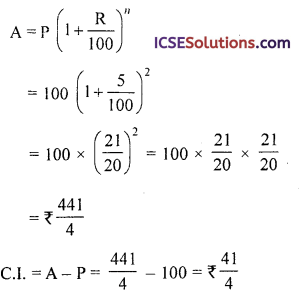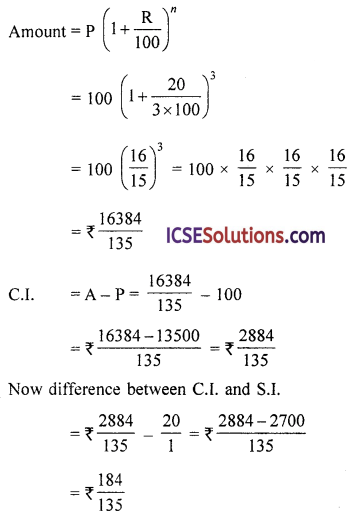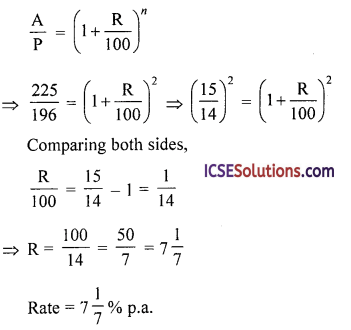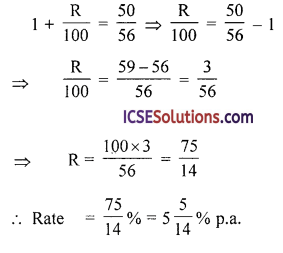Students appreciate clear and concise OP Malhotra Class 9 Solutions Chapter 2 Compound Interest Ex 2(C) that guide them through exercises.

## S Chand Class 9 ICSE Maths Solutions Chapter 2 Compound Interest Ex 2(C)

Question 1.
What amount of money should Mohan invest in a bank in order to get ₹ 1323 in 2 years at 5% compounded annually?
Solution:
Amount after 2 years = ₹ 1323
Rate (R) = 5% p.a.
Period = 2 years
Now A = P $$\left(1+\frac{\mathrm{R}}{100}\right)^n$$
⇒ ₹ 1323 = P $$\left(1+\frac{5}{100}\right)^2$$
⇒ ₹ 1323 = P $$\left(\frac{21}{20}\right)^2$$
⇒ P = ₹ 1323 x $$\left(\frac{20}{21}\right)^2$$
= 1323 x $$\frac { 20 }{ 21 }$$ x $$\frac { 20 }{ 21 }$$ = ₹ 1200
∴ Principal = ₹ 1200

Question 2.
Find the sum which amounts to ₹ 1352 in 2 years at 4% compound interest.
Solution:
Amount after 2 years = ₹ 1352
Rate (R) = 4% p.a.
Now A = P $$\left(1+\frac{\mathrm{R}}{100}\right)^n$$
⇒ ₹ 1352 = P $$\left(1+\frac{4}{100}\right)^2$$
⇒ ₹ 1352 = P x $$\left(\frac{26}{25}\right)^2$$
⇒ P = ₹ 1352 x ($$\frac { 25 }{ 26 }$$)²
= ₹ 1352 x $$\frac { 25 }{ 26 }$$ x $$\frac { 25 }{ 26 }$$ = ₹ 1250
∴ Principal = ₹ 1250Question 3.
What principal will amount to ₹ 9768 in two years. If the rates of interest for the successive years are 10% p.a. and 11% p.a. respectively.
Solution:
Amount after 2 years = ₹ 9768
Rate of interest for the first year (r1) = 10% p.a.
and for the second year (r2) = 11% p.a.
Now A = P $$\left(1+\frac{r_1}{100}\right)\left(1+\frac{r_2}{100}\right)$$
⇒ 9768 = P $$\left(1+\frac{10}{100}\right)\left(1+\frac{11}{100}\right)$$
⇒ 9768 = P $$\left(\frac{11}{10}\right)\left(\frac{111}{100}\right)$$
∴ P = ₹ 9768 x $$\frac { 10 }{ 11 }$$ x $$\frac { 100 }{ 11 }$$= ₹ 8000
∴ Principal = ₹ 8000

Question 4.
On what sum of money does the difference between the simple interest and compound interest in 2 years at 5% per annum is Rs. 15?
Solution:
Let principal (P) = ₹ 100
Rate (R) = 5% p.a.
Period in) = 2 years
Difference between S.I. and C.I. = ₹ 15
Now S.I = $$\frac{\mathrm{PRT}}{100}=\frac{100 \times 5 \times 2}{100}$$ = ₹ 10
and by compound interestNow difference between S.I. and C.I. = $$\frac { 41 }{ 4 }$$ – 10
= ₹ $$\frac{41-40}{4}=₹ \frac{1}{4}$$
If difference is ₹ $$\frac { 1 }{ 4 }$$, then principal = ₹ 100
If difference is ₹ 1, then principal = ₹$$\frac { 100×4 }{ 1 }$$
and if difference is ₹ 15, then principal
= ₹ $$\frac { 100×4×15 }{ 1 }$$ = ₹ 6000
Hence principal = ₹ 6000

Question 5.
The difference between simple and compound interest on the same sum of money at 6$$\frac { 2 }{ 3 }$$% for 3 years is ₹ 184. Determine the sum.
Solution:
Difference between C.I. and S.I. = ₹ 184
Let principal (Sum) = Rs. 100
Rate (R) = 6$$\frac { 2 }{ 3 }$$% = $$\frac { 20 }{ 3 }$$ %
Period (n) = 3 years
Simple interest = $$\frac{\mathrm{PRT}}{100}=\frac{100 \times 20 \times 3}{100 \times 3}$$
= ₹ 20
and by compound interestIf difference is ₹$$\frac { 184 }{ 135 }$$, then principal = ₹ 100
If difference is ₹ 1 then principal
= $$\frac{100 \times 135}{184}$$
and if difference is ₹ 184, then principal
= $$\frac{100 \times 135 \times 184}{184}$$ = ₹ 13500Question 6.
On what sum of money will the difference between the simple interest and the compound interest for 2 years at 5% per annum be equal to ₹ 50.
Solution:
Let sum (P) = ₹ 100
Rate (R) = 5% p.a.
Period (n) = 2 years
∴ Simple Interest = $$\frac { PRT }{ 100 }$$
= $$\frac{100 \times 5 \times 2}{100}$$ = ₹ 10
By compound interest,If difference is ₹$$\frac { 1 }{ 4 }$$, then principal = ₹ 100
and if difference is ₹ 1, then principal
= $$\frac { 100×4 }{ 1 }$$
and if difference is ₹ 50, then principal
= $$\frac{100 \times 4 \times 50}{1}$$ = ₹ 20,000

Question 7.
Find the rate per cent per annum, if compounded yearly
(i) Principal = ₹ 196, Amount = ₹ 225, time = 2 years
(ii) Principal = ₹ 3136, Compound interest = ₹ 345, Time = 2 years
Solution:
(i) Principal (P) = ₹ 196
Amount (A) = Rs. 225
Time = 2 years
We know that,(ii) Principal (P) = 136
C.I. = Rs. 345
∴ Amount (A) = P + C.I. = ₹ 3136 + 345 = ₹ 3481
Time (n) = 2 years
We know that,
Comparing, we getQuestion 8.
Hari purchased Relief Bonds for ^1000, a sum which will fetch him ₹ 2000 after 5 years. Find the rate of interest if the interest is compounded half-yearly.
(Given that $$\sqrt{2}$$ = 1.072)
Solution:
Principal (P) = ₹ 1000
Amount (A) = ₹ 2000
Period (n) = 5 years = 10 half years
We know that,
$$\frac{\mathrm{A}}{\mathrm{P}}=\left(1+\frac{\mathrm{R}}{100}\right)^n \Rightarrow \frac{2000}{1000}=\left(1+\frac{\mathrm{R}}{100}\right)^{10}$$
⇒ $$\frac{2}{1}=\left(1+\frac{R}{100}\right)^{10}$$
1 + $$\frac { R }{ 100 }$$ = $$\sqrt{2}$$ = 1.072
$$\frac { R }{ 100 }$$ = 1.072 – 1.000 = 0.072
R = 0.072 x 100 = 7.2
∴ Rate half-yearly = 7.2%
and rate annually = 7.2 x 2 = 14.4% p.a.

Question 9.
₹ 8000 became ₹ 9261 in a certain interval of time at the rate of 5% per annum C.l. Find the time.
Solution:
Principal (P) = ₹ 8000
Amount (A) = ₹ 9261
Rate (R) = 5% p.a.
We know that,
$$\frac{\mathrm{A}}{\mathrm{P}}=\left(1+\frac{\mathrm{R}}{100}\right)^n \Rightarrow \frac{9261}{8000}=\left(1+\frac{5}{100}\right)^n$$
⇒ $$\frac{9261}{8000}=\left(\frac{21}{20}\right)^n \Rightarrow\left(\frac{21}{20}\right)^{30}=\left(\frac{21}{20}\right)^n$$
Comparing, we get n = 3
∴ Period = 3 years

Question 10.
In how many years will a sum of ₹ 3000 at 20% per annum compounded semi-annually become ₹ 3993.
Solution:
Principal (P) = ₹ 3000
Amount (A) = ₹ 3993
Rate (R) = 20% p.a. or 10% half-yearly
We know that,
$$\frac{\mathrm{A}}{\mathrm{P}}=\left(1+\frac{\mathrm{R}}{100}\right)^n \Rightarrow \frac{3993}{3000}=\left(1+\frac{10}{100}\right)^n$$
⇒ $$\frac{1331}{1000}=\left(\frac{11}{10}\right)^n \Rightarrow\left(\frac{11}{10}\right)^3=\left(\frac{11}{10}\right)^n$$
Comparing, we get n = 3
∴ Period = 3 half years or 1 $$\frac { 1 }{ 2 }$$ yearsQuestion 11.
A sum of money put out at compound interest amounts in 2 years to ₹ 578.40 and in 3 years to ₹ 614.55. Find the rate of interest.
Solution:
Amount for 3 years = ₹ 614.55
Amount for 2 years = ₹ 578.40
Subtracting,
Interest for 1 year = ₹ 36.15
∴ ₹ 36.15 is interest on ₹ 578.40 for 1 year
∴ Rate = $$\frac{\text { Simple interest } \times 100}{\mathrm{P} \times \text { Time }}$$
= $$\frac{36.15 \times 100}{578.40 \times 1}=\frac{25}{4} \%=6 \frac{1}{4} \% \text { p.a. }$$

Question 12.
A sum compounded annually becomes $$\frac { 25 }{ 16 }$$ times of itself in 2 years. Determine the rate of interest per annum?
Solution:
Let Principal (P) = ₹ 1
Then Amount (A) = ₹ $$\frac { 25 }{ 16 }$$
Period (n) = 2 years
Let R be the rate of interest per annum, then
$$\frac{\mathrm{A}}{\mathrm{P}}=\left(1+\frac{\mathrm{R}}{100}\right)^n$$
$$\frac{25}{16}=\left(1+\frac{\mathrm{R}}{100}\right)^2 \Rightarrow\left(\frac{5}{4}\right)^2=\left(1+\frac{\mathrm{R}}{100}\right)^2$$
Comparing, we get
1 + $$\frac{\mathrm{R}}{100}=\frac{5}{4} \Rightarrow \frac{\mathrm{R}}{100}=\frac{5}{4}-1=\frac{5-4}{4}=\frac{1}{4}$$
∴ R = $$\frac { 1 }{ 4 }$$ x 100 = 25
∴ Rate = 25 % p.a.